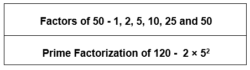# Factors Of 50

Factors of 50 are the numbers, the numbers which divide 50 completely, without leaving any remainder. All composite numbers have factors more than two. To find the factors of a number 50, we will use here simple multiplication method. The multiples of 50 are 50, 100, 150, 200, 250, 300, 350, 400, 450 and so on.## Factors Of 50 in Pairs

We can find the factors of number 50, by multiplying two numbers in a pair to get the original number as 50, such as;

1 × 50 = 50

2 × 25 = 50

5 × 10 = 50

Therefore,

 Factors Of 50 1, 2, 5, 10, 25 and 50

We can also write the negative pair factors of 50 as the product of two, to get the original number, such as:

-1 × -50 = 50

-2 × -25 = 50

-5 × -10 = 50

## How to Calculate Factors of 50?

To find the factors of 50, we have to check the natural numbers from 1 to 50. The numbers which can divide 50 completely or evenly, are the required factors.

50 ÷ 1 = 50

50 ÷ 2 = 25

50 ÷ 5 = 10

50 ÷ 10 = 5

50 ÷ 25 = 2

50 ÷ 50 = 1

## Prime Factorisation of 50

The number 50 is a composite number. Now let us find the prime factors of 50.

• The first step is to divide the number 50 with the smallest prime factor,i.e. 2.

50 ÷ 2 = 25

• Again, dividing 25 by 2, we get a fraction number 12.5, which is not considered as a factor.
• Now, proceed to the next prime numbers, i.e. 3, 5, 7 and so on

25 ÷ 3 = 8.33, 3 is not a factor of 50

• Divide 25 by 5.

25 ÷ 5 = 5

So, 5 is a factor of 50.

• Now, again by dividing 5 by 5 we get,

5 ÷ 5 = 1

• We have received 1 at the end and further, we cannot proceed with the division method. So, the prime factors of 50 are 2 × 5 × 5 or 2 × 52, where 2 and 5 are the prime numbers.

## Video Lesson on Prime Factors## Solved Examples

Q.1: If there are 50 pencils distributed equally in 5 boxes. What is the total number of pencils?

Solution: Given,

Number of pencils = 50

Number of boxes = 5

Number of pencils in each box = 50/5 = 10

Q.2: If the price of one mango is Rs. 5, then what is the cost of 10 mangoes?

Solution: Price of one mango = Rs. 5

Price of 10 mangoes = 10 x 5 = Rs. 50

Q.3: What are the common factors of 50 and 100?

Answer: Factors of 50 are 1,2,5,10,25 and 50

We know, 50 x 2 = 100

So, all the factors 50 are the factors of 100, excluding 100 itself.

Thus, 1,2,5,10,25 and 50 are common factors of 50 and 100.

## Frequently Asked Questions – FAQs

Q1

### What are the factors of 50?

The factors of 50 are the natural numbers that divide the original number, evenly. They are 1,2,5,10,25 and 50.
Q2

### Are the factors and multiples of 50, same or different?

Factors of 50 are different from multiples of 50. Factors divide the original number into equal parts whereas multiples are the product form of number when multiplied by different natural numbers.
Q3

### What are the multiples of 50 in Maths?

The first ten multiples of 50 are 50, 100, 150, 200, 250, 300, 350, 400, 450 and 500.
Q4

### Which is the highest prime factor of 50?

By prime factorisation of 50, we know that, 50 = 2 x 5 x 5. Therefore, the greatest prime factor of 50 is 5.
Q5

### What are common factors of 50, 125 and 150?

Factors of 50 = 1, 2, 5, 10, 25, 50
Factors of 125 = 1, 5, 25, 125
Factors of 175 = 1, 2, 3, 5, 6, 10, 15, 25, 30, 50, 75, 150
Common factors of 50, 125 and 175 = 1, 5 and 25
 Links Related to Factors Factors of 15 Factor of 36 Factors of 48 Factors of 18 Factors of 42 Factors of 60 Factors of 35 Factors of 27 Factors And Multiples
Test your knowledge on Factors of 50